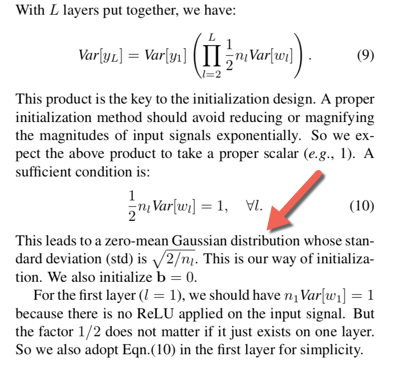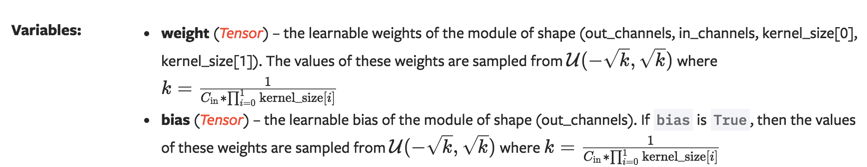# Kaiming init of conv and linear layers, why gain = sqrt(5) #15314

Closed
opened this issue Dec 17, 2018 · 3 comments
Closed

# Kaiming init of conv and linear layers, why gain = sqrt(5)#15314

opened this issue Dec 17, 2018 · 3 comments

###mratsim commented Dec 17, 2018 • edited

cc @fmassa as he introduces those in #9038.

Looking into the initialisation of Linear and Convolution layers we have the following

Linear:

Lines 58 to 63 in 3df79f4

 def reset_parameters(self): init.kaiming_uniform_(self.weight, a=math.sqrt(5)) if self.bias is not None: fan_in, _ = init._calculate_fan_in_and_fan_out(self.weight) bound = 1 / math.sqrt(fan_in) init.uniform_(self.bias, -bound, bound)

Convolution:

Lines 45 to 51 in 3df79f4

 def reset_parameters(self): n = self.in_channels init.kaiming_uniform_(self.weight, a=math.sqrt(5)) if self.bias is not None: fan_in, _ = init._calculate_fan_in_and_fan_out(self.weight) bound = 1 / math.sqrt(fan_in) init.uniform_(self.bias, -bound, bound)

Notice the sqrt(5) scaling factor.

## Kaiming paper

https://arxiv.org/abs/1502.01852

The standard deviation should be sqrt(2 / fan_in)Using the same principle as Glorot et al paper, for an uniform distribution we should use bounds of ±√3 * sqrt(2 / fan_in)

This is what is done here:

Lines 288 to 293 in 700271d

 fan = _calculate_correct_fan(tensor, mode) gain = calculate_gain(nonlinearity, a) std = gain / math.sqrt(fan) bound = math.sqrt(3.0) * std # Calculate uniform bounds from standard deviation with torch.no_grad(): return tensor.uniform_(-bound, bound)

## Diving deeper into the implementation

It seems like the a = √5 is used in

Lines 8 to 47 in 700271d

 def calculate_gain(nonlinearity, param=None): r"""Return the recommended gain value for the given nonlinearity function. The values are as follows: ================= ==================================================== nonlinearity gain ================= ==================================================== Linear / Identity :math:1 Conv{1,2,3}D :math:1 Sigmoid :math:1 Tanh :math:\frac{5}{3} ReLU :math:\sqrt{2} Leaky Relu :math:\sqrt{\frac{2}{1 + \text{negative\_slope}^2}} ================= ==================================================== Args: nonlinearity: the non-linear function (nn.functional name) param: optional parameter for the non-linear function Examples: >>> gain = nn.init.calculate_gain('leaky_relu') """ linear_fns = ['linear', 'conv1d', 'conv2d', 'conv3d', 'conv_transpose1d', 'conv_transpose2d', 'conv_transpose3d'] if nonlinearity in linear_fns or nonlinearity == 'sigmoid': return 1 elif nonlinearity == 'tanh': return 5.0 / 3 elif nonlinearity == 'relu': return math.sqrt(2.0) elif nonlinearity == 'leaky_relu': if param is None: negative_slope = 0.01 elif not isinstance(param, bool) and isinstance(param, int) or isinstance(param, float): # True/False are instances of int, hence check above negative_slope = param else: raise ValueError("negative_slope {} not a valid number".format(param)) return math.sqrt(2.0 / (1 + negative_slope ** 2)) else: raise ValueError("Unsupported nonlinearity {}".format(nonlinearity))

The a is only used for leaky_relu, which actually is the default if we don't pass any activation to kaiming_uniform:

Line 261 in 700271d

 def kaiming_uniform_(tensor, a=0, mode='fan_in', nonlinearity='leaky_relu'):

Furthermore this √5 factor conflicts with the recommended sqrt(2.0 / (1 + negative_slope ** 2)) in calculate_gains, and I suspect this is unintentional.

# Docs

Whether the √5 factor is intentional or not, the documentation is wrong for the weights.

## LinearWhile for bias k = 1/in_features is true, for the weight, k = 6/in_features assuming pure Kaiming, or k = 6 * 5/in_features at the moment.

## ConvolutionSame remark

## Closing thoughts

Plenty of tutorials uses ReLU and not LeakyReLU, having the default initialisation for kaiming_uniform to leaky relu would create suboptimal training for those.

At the very least it should be noted in the documentation that Linear and Conv layers initialisation is done assuming it is followed by a leaky relu activation.

Finally the √5 should be explained.

###eugeneware commented Dec 17, 2018 • edited

 I've also being trying to work out where the sqrt(5) factor comes from for Linear layer initialisation. This thread explains the reasoning. It was due to a refactor of initialisation code.

###soumith commented Mar 28, 2019

 closing via @eugeneware 's comment. the code refactor from jramseyer changes the default pytorch initialization from manually initializing the weights by calling random number generator function uniform to using torch.nn.init.kaiming -- but it wanted to have the same end-result in weights, because we wanted to preserve backward-compatibility. So the sqrt(5) is nothing more than giving the code the same end-result as before. The initialization itself comes from torch7 and torch5 and is a modified version of initialization fro Lecun'98 Efficient Backprop. This post gives more context: https://plus.google.com/106447253626219410322/posts/RZfdrRQWL6u